# JEE Height and Distance Previous Year Questions With Solutions

Height and distance is an important topic from competitive examination point of view. You must have seen the problems where the height of one tower is given, and then the top or bottom angles of another tower are given from the top of that tower, and you need to determine the height of the second tower. In this article, we will covered such problems to help students understand the concept well. Further, to help students understand the concepts clearly, we are also offering chapter wise solutions that consists of JEE previous year solved questions. Every solution of this study material is arranged in a systematic manner in order to give students an easy learning experience while solving the questions. Students are advised to download these solutions and practice to crack the JEE exams.

### Terms used in Heights and Distances:

 There are certain terms used while dealing with topic-height and distance which are described as follows: Ray of Vision: The ray from the eye of the observer towards the object under observation. Angle of Elevation: If the object under observation is above the horizontal ray passing through the point of observation, the measure of the angle formed by the horizontal ray and the ray of vision. Angle of Depression: If the object under observation is below the horizontal ray passing through the point of observation, the measure of the angle formed by the horizontal ray and the ray of vision.

## JEE Main Past Year Questions With Solutions on Height and Distance

Question 1: If the angles of elevation of the top of a tower from three collinear points A, B and C, on a line leading to the foot of the tower, are 300, 450 and 600 respectively, then the ratio AB:BC, is:

(a) 1: √3                 (b) 2:3                      (c) √3 : 1                     (4) √3 : √2

Solution: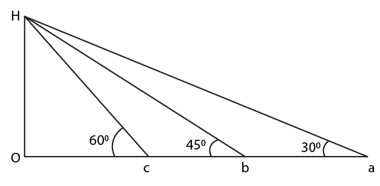Let OH = h

From triangle HOC, tan 600 = h/OC

=> OC = h/√3

From triangle, HOA, tan 300 = h/OA

=> OA = h√3

From triangle, HOB, tan 450 = h/OB

=> OB = h

Now, AB = OA – OB = h(√3 – 1)

BC = OB – OC = h(1 – 1/√3) = h/√3 (√3 – 1)

AB : BC = h(√3 – 1) : h/√3 (√3 – 1)

=> AB : BC = √3 : 1

Question 2: A tower subtends an angle α at a point on the same level as the foot of the tower and at the second point, b metres above the first, the angle of depression of the foot of the tower is β. The height of the tower is

(a) b cot α tan β

(b) b tan α tan β

(c) b tan α cot β

(d) None of these

Solution: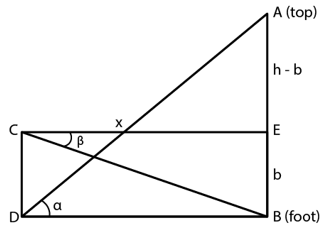From figure,

In right triangle, ABD,

AB/BD = tan α

h/x = tan α => h = x tan α

Again, from the right triangle, BCE,

BE/EC = tan β

=> b/x = tan β

=> x = b/(tan β), substitute in above equation, we get

h = b/(tan β) x tan α = b cot β tan α

Question 3: A vertical pole consists of two parts, the lower part being one third of the whole. At a point in the horizontal plane through the base of the pole and distance 20m from it, the upper part of the pole subtends an angle whose tangent is 1/2. The possible heights of the pole are –

(a) 20 m and 20√3 m

(b) 20 m and 60 m

(c) 16 m and 48 m

(d) None of these

Solution: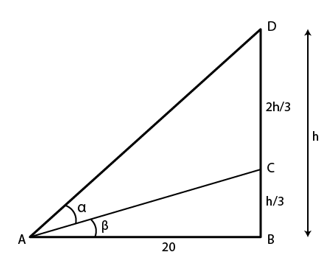In triangle, BAC

tan β = (h/3)/20 = h/60

tan(α + β) = h/20

[We know, tan(x + y) = [tan x – tan y]/[1 – tan x tan y]]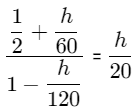[60+2h]/[120-h] = h/20

=> h2 – 80h + 1200 = 0

=> h = 20 m and 60 m

Question 4: An observer on the top of a tree finds the angle of depression of a car moving towards the tree to be 300. After 3 min this angle becomes 600. After how much more time will the car reach the tree

(a) 4 min                                (b)  1.5 min                           (c) 4.5 min                             (d) 2 min

Solution: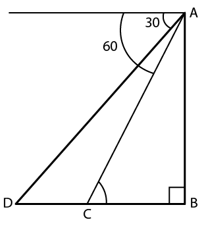Let x unit/min be the speed of car.

DC = 3x

Let h be the height.

Here angle ACB = 600 and angle ADB = 300

In right triangle ABC,

tan 60 = h/BC

h = √3 BC

In triangle,

tan 600 = h/BD

=> h = √3 BD

BC + CD = √3 h

h + 3x = √3 h

h = 3x/(√3 -1)

or h = 3x/(√3-1) x (√3+1)/

or h = [3x(√3+1)]/2

Time taken = distance/speed = BC/x = h/x

= [3x(√3+1)]/2x

= (1.5) (2.73)

= 4 min

Question 5: A house of height 100 m subtends a right angle at the window of an opposite house. If the height of the window is 64 m, then the distance between the two houses is

(a) 48 m                                (b) 36 m                               (c) 54 m                        (d) 72 m

Solution: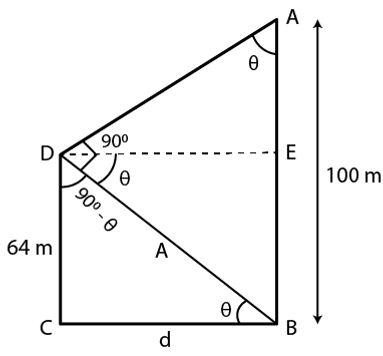In triangle BCD,

tan θ = 64/d

In triangle AED,

(100- 64) tan θ = d

=>36(64/d) = d

=> d2 = 36 x 64

=>d = 6×8 = 48 m

Question 6: A man is walking towards a vertical pillar in a straight path, at uniform speed. At a certain point A on the path, he observes that the angle of elevation of the top of the pillar is 300. After walking for 10 minutes from A in the same direction, at a point B, he observes that the angle of elevation of the top of the pillar is 600. Then the time taken (in minutes) by him, from B to reach the pillar, is?

(a) 5                                    (b) 6                                    (c) 10                                   (d) 20

Solution: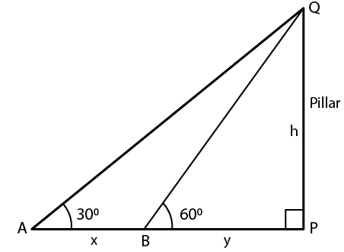In triangle QPB: tan 600 = h/y => h = √3 y

In triangle QPA: tan 300 = h/(x+y) => √3h = x + y

From above equations, we have

√3(√3 y) = x + y

3y = x + y

=> y = x/2

As speed is uniform,

To go with x, it takes around 10 min and with x/2, it takes around 5 min.

Question 7: The angle of elevation of a tower at a point distant d meters from its base is 300?. If the tower is 20 meters high, then the value of d is

(a) 10√3 m

(b) 20√3 m

(c) 10 m

(d) 20/√3 m

Solution:

20 cot 300 = d

=> d = 20√3 m

Question 8: Let a vertical tower AB have its end A on the level ground. Let C be the mid-point of AB and P be a point on the ground such that AP = 2AB. If ∠BPC = β, then tanβ is equal to.

(a) 1/4                               (b) 6/7                            (c) 1/4                             (d) 2/9

Solution: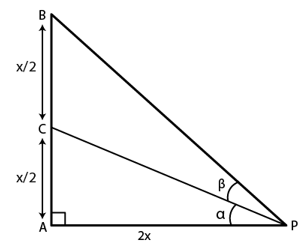Let AB = x, then AP = 2AB = 2x

In right triangle ABP,

BP2 = AP2 + AB2

BP2 = (2x)2 + x2 = 5x2

=> BP = √5 x

Also, AC = x/2

and tan α = (x/2)/2x = 1/4

tan(α + β) = x/2x = 1/2

Now,

$\frac{tan \alpha + tan \beta}{1 – tan \alpha \; tan \beta} = \frac{1}{2}$

=> 2(tanα + tanβ) = 1 – tanα tanβ

=> 2(1/4 + tanβ) = 1 – (1/4) tanβ

Solving above equation, we get

tan β = 2/9

Question 9: PQR is a triangular park with PQ = PR = 200 m. A T.V. tower stands at the mid-point of QR. If the angles of elevation of the top of the tower at P, Q and R are respectively 450, 300 and 300, then the height of the tower (in m) is,

(a) 50√2                        (b) 100                               (c) 70                            (d) 100√3

Solution: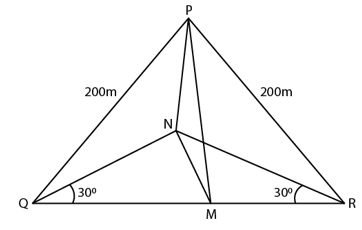In triangle MNQ,

tan 30 = MN/QM = h/QM = 1/√3

QM = √3 h = MR

In triangle PMQ,

(PQ)2 = (MP)2 + (MQ)2

2002 = h2 + (√3h) 2

or h = 100 m

Question 10: The angle of elevation of the top of a tower from the top and bottom of a building of height a are 30° and 45° respectively. If the tower and the building stand at the same level, then what is the height of the tower?

Solution: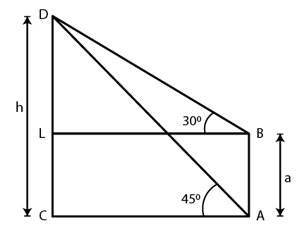Here CD = Tower of height h and AB = building of height a

In right triangle BLD, tan 300 = (h-a)/LB

=> LB = (h-a)/tan 300 = √3(h – a)

From right triangle ACD, tan 450 = h/AC

Here AC = LB

Or h(√3 – 1) = √3 a

or h = √3a/(√3-1) = [√3a(√3+1)]/2

Therefore, h = [(3 + √3)a]/2

Other Important Questions:

Question 11: At a distance 2h from the foot of a tower of height h, the tower and a pole at the top of the tower subtend equal angles. Height of the pole should be,

(a) 5h/3                                     (b) 4h/3                       (c) 7h/5                           (d) 3h/2

Solution:

Let α be the angle and h be the height of the pole.

tan α = 1/2 and tan 2α = (p+h)/2h

we know, tan 2α = 2tanα/(1-tan2α)

=> (p + h)/2h = 1/(1-1/4)

=> (p + h)/2h = 4/3

=> p = 5h/3

Question 12: An aeroplane flying horizontally 1 km above the ground is observed at an elevation of 600 and after 10 seconds the elevation is observed to be 300. The uniform speed of the aeroplane in kmph is.

(a) 240                        (b) 240√3                     (c) 60√3                        (d) None of these

Solution: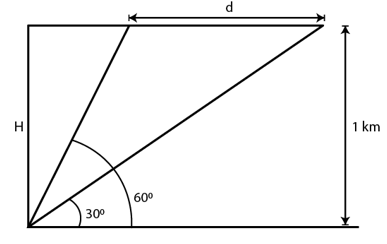d = H cot 300 – H cot 600

Time taken = 10 sec

So, speed = [cot300 – cot600]/10 x 60 x 60 = 240√3

Question 13: From a point a meter above a lake the angle of elevation of a cloud is α and the angle of depression of its reflection is β. Prove that height of the cloud is H = [a sin(α + β)]/[sin(β – α)].

Solution: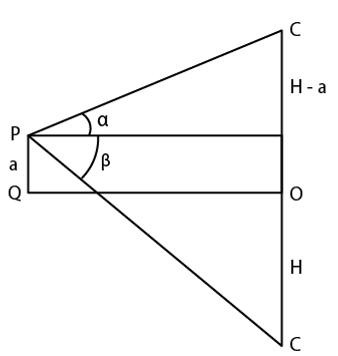(H + a) cotβ = (H – a) cot α

We know, cot α + cotβ = [sin(α + β)]/[sin α sinβ]

and cot α – cotβ = [sin(β – α)]/[sin α sinβ]

=> H = [a sin(α + β)]/[sin(β – α)]. Hence Proved.

Question 14:

A person, standing on the bank of a river observes that the angle subtended by a tree on the opposite bank is 600, when he retreats 40 m from the bank, he finds the angle to be 300 . The height of the tree and the breadth of the river are.

(a) 10√3 m and 10 m

(b) 20√3 m and 10 m

(c) 20√3 m and 20 m

(d) None of these

Solution: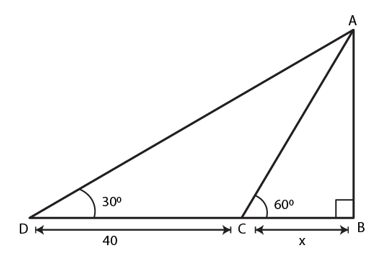Let AB= h be the height of the tree and CB = x, the breadth of the river.

Here angle BDA = 300

From right triangle, ABC,

tan 600 = AB/BC => h/x = √3

=> h = √3 x …..(i)

From right triangle, ABD,

tan 300 = AB/BD

=> 1/√3 = h/(40+x)

=> √3 h = 40 + x

Using (i), we get

(√3) √3x = 40 + x

=> 3x = 40 + x

or x = 20

(i)=> h = √3 x 20 = 20√3

Thus, height of the tree = 20√3 and

breadth of the river = 20 m

Test your Knowledge on Height and distance